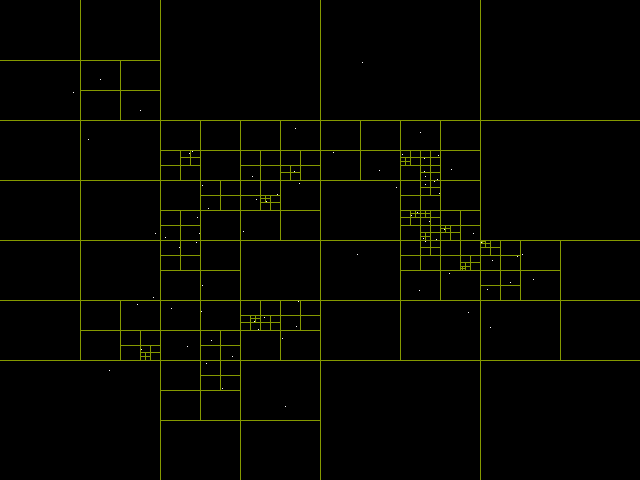# Amin Mesbah

## Debugging a Quadtree in C

Posted on 2017-05-12

I've been working on a project that simulates stars or particles and the forces of attraction between them. To make the simulation more efficient and able to handle more particles, I've been working on implementing a Barnes-Hut Algorithm by using a quadtree to partition the simulation space. When it works, it's beautiful:```//qt_test.c

#include <stdio.h>
#include <stdlib.h>

{
};

{
};

{
if (node)
{
node->ne = NULL;
node->nw = NULL;
node->sw = NULL;
node->se = NULL;
}
return node;
}

{
if (node)
{
free(node);
}
}

{
if (node && remaining_levels > 0)
{
remaining_levels--;

}
}

{
if (qt)
{
}
return qt;
}

{
if (qt)
{
free(qt);
}
}

void update(struct QuadTree *qt)
{
}

int main(void)
{

for (int i = 0; i < NUM_UPDATES; ++i)
{
printf("Update %d\n", i+1);
update(qt);
}

return 0;
}
```

Here's a sample from the output of `valgrind --track-origins=yes ./qt_test`:

``````Invalid read of size 8
==16345==    at 0x1088B7: quad_tree_node_free (qt_test.c:43)
==16345==    by 0x1089DB: quad_tree_free (qt_test.c:82)
==16345==    by 0x108A00: update (qt_test.c:90)
==16345==    by 0x108A5D: main (qt_test.c:102)
==16345==  Address 0x5e350a0 is 16 bytes inside a block of size 32 free'd
==16345==    at 0x4C2C14B: free (in /usr/lib/valgrind/vgpreload_memcheck-amd64-linux.so)
==16345==    by 0x1088D0: quad_tree_node_free (qt_test.c:45)
==16345==    by 0x1089DB: quad_tree_free (qt_test.c:82)
==16345==    by 0x108A00: update (qt_test.c:90)
==16345==    by 0x108A5D: main (qt_test.c:102)
==16345==  Block was alloc'd at
==16345==    at 0x4C2AF1F: malloc (in /usr/lib/valgrind/vgpreload_memcheck-amd64-linux.so)
==16345==    by 0x10885E: quad_tree_node_init (qt_test.c:25)
==16345==    by 0x1089A0: quad_tree_init (qt_test.c:71)
==16345==    by 0x108A30: main (qt_test.c:97)

[...]

==16345== Invalid free() / delete / delete[] / realloc()
==16345==    at 0x4C2C14B: free (in /usr/lib/valgrind/vgpreload_memcheck-amd64-linux.so)
==16345==    by 0x1088D0: quad_tree_node_free (qt_test.c:45)
==16345==    by 0x1088BF: quad_tree_node_free (qt_test.c:43)
==16345==    by 0x1089DB: quad_tree_free (qt_test.c:82)
==16345==    by 0x108A00: update (qt_test.c:90)
==16345==    by 0x108A5D: main (qt_test.c:102)
==16345==  Address 0x5e35570 is 0 bytes inside a block of size 32 free'd
==16345==    at 0x4C2C14B: free (in /usr/lib/valgrind/vgpreload_memcheck-amd64-linux.so)
==16345==    by 0x1088D0: quad_tree_node_free (qt_test.c:45)
==16345==    by 0x1088BF: quad_tree_node_free (qt_test.c:43)
==16345==    by 0x1089DB: quad_tree_free (qt_test.c:82)
==16345==    by 0x108A00: update (qt_test.c:90)
==16345==    by 0x108A5D: main (qt_test.c:102)
==16345==  Block was alloc'd at
==16345==    at 0x4C2AF1F: malloc (in /usr/lib/valgrind/vgpreload_memcheck-amd64-linux.so)
==16345==    by 0x10885E: quad_tree_node_init (qt_test.c:25)
==16345==    by 0x10890B: quad_tree_node_subdivide (qt_test.c:54)
==16345==    by 0x108958: quad_tree_node_subdivide (qt_test.c:61)
==16345==    by 0x1089B0: quad_tree_init (qt_test.c:72)
==16345==    by 0x108A30: main (qt_test.c:97)
``````

I was baffled for quite a while by these errors. I spent a lot of time checking and re-checking all the functions that init and free the quadtree and quadtree nodes. Only after expending great effort did I finally determine the cause.

The problem turned out to lie with the way I was passing my struct around to different functions. I'm used to C++, in which one can pass things either by value or by reference, but in C, everything is passed by value. My `update()` function was changing its local copy of the `QuadTree *`, `qt`, but that change did not apply to the original `qt` in `main()`. That meant that all the calls to `qt` in `main()` were accessing the old memory that had been freed.

### Another Level of Indirection

The first solution I found was to change `update()` to take a `QuadTree **` parameter:

```void update(struct QuadTree **qt)
{
}
```

Then in `main()` I called it like:

```update(&qt);
```

Now that the `qt` parameter was wrapped in an extra pointer, changes to it in `update()` were now being properly applied to `qt` in `main()`. Valgrind's previous complaints were gone. It was a nice example to support the claim that "All problems in computer science can be solved by another level of indirection."

### Change Only What Needs Changing

I also found another way of solving the problem. I already had a `QuadTree` struct which conveniently wrapped the rest of the actual tree and kept track of the root. I realized that there was no reason to continually be freeing and reallocating `qt` itself.

I simplified the `QuadTree` functions to basically be "run-once".

```struct QuadTree* quad_tree_init(void)
{
qt->root = NULL;
return qt;
}
```
```void quad_tree_free(struct QuadTree *qt)
{
if (qt)
{
free(qt);
}
}
```

I changed my update function to only operate on `qt->root`.

```void update(struct QuadTree *qt)
{
}
```

And I updated my main function accordingly:

```int main(void)
{

for (int i = 0; i < NUM_UPDATES; ++i)
{
printf("Update %d\n", i+1);
update(qt);
}

Still no errors from Valgrind! `qt` is happy to point to the same memory address for most of the program.